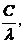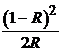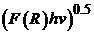﻿ Undergraduate Experiment Using Absorption and Diffuse Reflectance Spectroscopies: Theoretical and Experimental Bandgap Calculations of Porphyrins and MetalloporphyrinsPublications are Open
Access in this journal
Article Versions
Export Article
• Normal Style
• MLA Style
• APA Style
• Chicago Style
Research Article
Open Access Peer-reviewed

### Undergraduate Experiment Using Absorption and Diffuse Reflectance Spectroscopies: Theoretical and Experimental Bandgap Calculations of Porphyrins and Metalloporphyrins

Muna Bufaroosha , Shaikha S. Al Neyadi, Ahmed Alzamly, Mohamad Toutounji, Na’il Saleh, Bashar Yousef Abuhattab, Abdullah Al-Hemyari, Amna Alyammahi, Shamma Alzahmi, Mohamed Altubji, Ruba Al-Ajeil
World Journal of Chemical Education. 2020, 8(2), 87-91. DOI: 10.12691/wjce-8-2-4
Received March 09, 2020; Revised April 16, 2020; Accepted April 22, 2020

### Abstract

In this work, an undergraduate-level experiment using 5,10,15,20-tetraphenylporphyrin (H2TPP) and its corresponding Ni(II) and Zn(II) metal complexes was developed. The bandgaps of H2TPP and its Ni(II) and Zn(II) metal complexes were calculated experimentally and theoretically to evaluate their abilities to act as light-harvesting complexes.

### 1. Introduction

In photosynthesis, light-harvesting complexes (LHCs) act as antennas that absorb light energy of a specific wavelength and pass this energy to the reaction center, where the charge is isolated and the energy is stored as chemical energy 1. Plants employ this process very efficiently in photosynthesis. During photosynthesis, photosynthetic molecules are active in the range of 400–700 nm; that is, in the visible light region. Visible light represents 43% of the solar energy reaching the Earth. Natural LHCs are composed of a chromophore and protein base. The chromophore is where the light energy is stored in the form of the electronic excited state of the molecule and then transferred to the reaction center. The protein-based component of the LHC acts as a scaffold that regulates the arrangements of the chromophores and adjusts their photochemical behavior 2.

Inspired by nature, here we investigate some synthetic porphyrins as potential LHCs. Our interest in porphyrins stems from the fact that they have close structural resemblance to chlorophylls. Each chlorophyll molecule has a porphyrin ring with a magnesium(II) ion in its center (head group) and a hydrophobic tail. Porphyrins can efficiently absorb visible light 3. Porphyrins are also capable of absorbing energy in other regions such as the near-infrared and infrared regions depending on their structure. The unique chemical structures of porphyrins make them interesting molecules to tune for a specific photochemical process. Because porphyrins contain conjugated macrocyclic rings, they display excellent electron-donating ability and long-lived excited states. For instance, tetrasodium meso-tetra(sulfonatophenyl)porphine exhibits a lifetime of 11.75 ns in neutral solution 4.

The ability of a complex to behave as an LHC can be evaluated by measuring its bandgap energy (Eg). A small Eg of around 2.0 eV between the highest occupied molecular orbital (HOMO) and lowest unoccupied molecular orbital (LUMO) energy levels of the complex is preferred for the energy transfer process in the visible region. For example, chlorophyll a has an Eg of 2.357 eV and absorption peaks at 420 and 670 nm 5.

In this experiment, 5,10,15,20-tetraphenylporphyrin (H2TPP) (1), and its corresponding Ni(II) and Zn(II) metal complexes NiTPP (2) and ZnTPP (3), respectively, are synthesized and their Eg are calculated experimentally via ultraviolet–visible (UV-Vis) absorption spectroscopy and diffuse reflectance spectroscopy (DRS). Furthermore, Eg values determined from theoretical calculations using Q-Chem 5.1 are compared with experimentally obtained values.

### 2. Materials and Methods

2.1. Materials

All chemicals were purchased from Sigma Aldrich and used as received without further purification. Compound 1, 2, and 3 were synthesized and characterized as described previously 6.

2.2. UV-Vis Measurements

UV-Vis spectra were recorded using a UV-Vis spectrophotometer (UV-3600, Shimadzu, Japan) at room temperature in quartz cells with a pathlength of 1 cm in the range of 200–700 nm. To prepare stock solutions, proper amounts of each compound were dissolved in dichloromethane (CH2Cl2) to obtain a concentration of 10−6 M. Optical Eg values were measured for solid-state samples obtained by mixing each sample (2.00 mg) with BaSO4 (3.18 g) as a reference material.

2.3. Computational Methods

Quantum chemical calculations were carried out using Q-Chem 5.1 7. The ab initio calculations were based on time-dependent density functional theory (TD-DFT). The calculations were performed using the B3LYP exchange correlation functional with the 6-31G basis set, zero charge, and spin multiplicity of one. The point group of 1 was assumed to be C2v and that of 2 and 3 was assumed to be D2d.

### 3. Results and Discussion

3.1. Experimental Bandgap Measurement
3.1.1. Bandgap Measurement Using UV-Vis Absorption Spectroscopy

The UV-Vis absorption spectra of compound 1, 2, and 3 were measured in CH2Cl2 in the region of 200–700 nm. Table 1 lists the Soret and Q bands of the three compounds.

• Figure 1. (A) Absorption spectrum of 1 in CH2Cl2 (1×10−6 M). (B) Tauc/Davis–Mott plot used to determine the bandgap energy (Eg) of 1. (C) Absorption spectrum of 2 in CH2Cl2 (1×10−6 M). (D) Tauc/Davis–Mott plot used to determine Eg of 2. (E) Absorption spectrum of 3 in CH2Cl2 (1×10−6 M). (F) Tauc/Davis–Mott plot used to determine Eg of 3

The Eg values of compound 1, 2, and 3 were resolved using the curve-fitting method of the UV-Vis spectra according to the Tauc/Davis–Mott model 8. This method uses the equation 𝛼h𝜈=K(h𝜈−Eg)1⁄2, where 𝛼 is the absorption coefficient, h𝜈 is the incident photon energy, and K is an energy-independent constant. A plot of (𝛼h𝜈)2 versus h𝜈 derived from the UV-Vis data allows Eg to be obtained by extrapolating a line through the steep linear part of the curve to the energy axis. From the absorption spectra, the wavelength was converted to energy and used to calculate α. In addition, Eg=hwhere h is Planck’s constant (6.62×1034 J.s), C is the speed of light (2.999×108 m/s), and λ is the wavelength of incident photons. By converting energy from J to eV, the Tauc equation can be rewritten as (αhν)2=(2.303×A× hν)2, where A is the absorbance. Subsequently, a plot of the x-axis as the energy in eV and y-axis as (αhv)2, as shown in Figure 1(B, D, and F), allows Eg to be estimated from the tangent line on the curve of the plot.

3.1.2. Bandgap Measurement Using Diffuse Reflectance Spectroscopy

A UV–Vis absorption spectrum provides information about the electronic transitions between the different orbitals of a solid. The increase of absorbance at a certain wavelength is regarded as an indication of electron excitation from the valence band to the conduction band. In the Kubelka–Munk method, the equation F(R)=is used. In this equation, F(R) is assumed to be equal to α and R is the reflectance of the material. Whenis plotted against the energy in eV, the intercept of the straight part of the curve is regarded as Eg in eV, as demonstrated in Figure 2(B, D, and F) 9.

3.2. Computational Method to Evaluate Bandgap

Our calculations showed that the stable geometry of 1 was the C2v point group and that of 2 and 3 was the D2d point group. To evaluate the absorption spectra, the energies of the excited states of the complexes were calculated using the Energy/TD-DFT, Basis 6-31G, and Exchange B3LYP functions. The ground and excited states of the three materials were calculated in the gas phase by using the Hartee–Fock and TD-DFT methods, respectively. Calculations considering solvent effects were not performed. Three methods were used to evaluate Eg of 13. The results are summarized in Table 2. Figure 3, Figure 4, and Figure 5 present the results of the theoretical Eg calculations of 1, 2, and 3, respectively.

• Figure 2. (A) DRS profile of 1. (B) Kubelka–Munk plot used to determine the bandgap (Eg) of 1. (C) DRS profile of 2. (D) Kubelka–Munk plot used to determine Eg of 2. (E) DRS profile of 3. (F) Kubelka–Munk plot used to determine Eg of 3
• Figure 3. Energy gap of 1 calculated using QCHEM software
• Figure 4. Energy gap of 2 calculated using QCHEM software
• Figure 5. Energy gap of 3 calculated using QCHEM software

The insertion of a transition metal into the cavity of the porphyrin aligned the phenyl rings on the periphery of the porphyrin ring, which increased the symmetry of the metalloporphyrins (2 and 3) over that of 1. Our theoretical results suggested that the larger Eg values of 2 and 3 than those of 1 could stem from the interaction of the metals with the frontier orbitals of the porphyrin ligand. The obtained Eg values support the potential of 1-3 as LHCs. The notable differences between the Eg values obtained from experimental measurements and theoretical calculations may be because the theoretical calculations were performed in the gas phase.

### 4. Conclusion

Experimental and theoretical methods can be used to evaluate Eg of porphyrins. Here we determined Eg of porphyrin 1 and metalloporphyrin 2 and 3 using UV-Vis absorption spectroscopy, DRS, and ab initio calculations. Based on our results, the investigated compounds could serve as LHCs because they showed photoactivity in the UV-Vis range.

### Acknowledgments

We thank the undergraduate students that helped with confirming the theoretical calculations. We also acknowledge the students Layla Al Mazroui and Shahira Hussein Adib.

### References

  Shah, E.V., et al., Co-Tetraphenylporphyrin (co-TPP) in TM-TPP (TM = Fe, Co, Ni, Cu, and Zn) series: a new optical material under DFT. Journal of Molecular Modeling, 2018. 24(9), 239. In article View Article  PubMed  Alzamly, A., Alawadhi, A., Ahmed, S., Bakiro, M., & Bufaroosha, M., Photosensitizer Using Visible Light: An Undergraduate Laboratory Experiment Utilizing an Affordable Photocatalytic Reactor. International Journal for Innovation Education and Research, 2018. 6(11), 74-84. In article View Article  Penttilä, A., C.R. Boyle, and M.L. Salin, Active Oxygen Intermediates and Chlorophyllin Bleaching. Biochemical and Biophysical Research Communications, 1996. 226(1), 135-139. In article View Article  PubMed  Zhao, Q., et al., Out-of-Plane Coordinated Porphyrin Nanotubes with Enhanced Singlet Oxygen Generation Efficiency. Scientific Reports, 2016. 6(1), 31339. In article View Article  PubMed  Tauc, J., Absorption edge and internal electric fields in amorphous semiconductors. Materials Research Bulletin, 1970. 5(8), 721-729. In article View Article  Neyadi, S.S.A., et al., An Undergraduate Experiment Using Microwave-Assisted Synthesis of Metalloporphyrins: Characterization and Spectroscopic Investigations. World Journal of Chemical Education, 2019. 7(1), 26-32. In article View Article  Kong, J., et al., Q-Chem 2.0: a high-performance ab initio electronic structure program package. Journal of Computational Chemistry, 2000. 21(16), 1532-1548. In article  Li, X., et al., Determination of band gaps of self-assembled carbon nanotube films using Tauc/Davis–Mott model. Applied Physics A, 2009. 97(2), 341-344. In article View Article  López, R. and R. Gómez, Band-gap energy estimation from diffuse reflectance measurements on sol–gel and commercial TiO2: a comparative study. Journal of Sol-Gel Science and Technology, 2012. 61(1), 1-7. In article View Article

Published with license by Science and Education Publishing, Copyright © 2020 Muna Bufaroosha, Shaikha S. Al Neyadi, Ahmed Alzamly, Mohamad Toutounji, Na’il Saleh, Bashar Yousef Abuhattab, Abdullah Al-Hemyari, Amna Alyammahi, Shamma Alzahmi, Mohamed Altubji and Ruba Al-AjeilThis work is licensed under a Creative Commons Attribution 4.0 International License. To view a copy of this license, visit http://creativecommons.org/licenses/by/4.0/